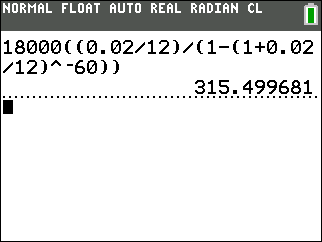# 5.5: Loans

•• Maxie Inigo, Jennifer Jameson, Kathryn Kozak, Maya Lanzetta, & Kim Sonier
• Coconino Community College
$$\newcommand{\vecs}{\overset { \rightharpoonup} {\mathbf{#1}} }$$ $$\newcommand{\vecd}{\overset{-\!-\!\rightharpoonup}{\vphantom{a}\smash {#1}}}$$$$\newcommand{\id}{\mathrm{id}}$$ $$\newcommand{\Span}{\mathrm{span}}$$ $$\newcommand{\kernel}{\mathrm{null}\,}$$ $$\newcommand{\range}{\mathrm{range}\,}$$ $$\newcommand{\RealPart}{\mathrm{Re}}$$ $$\newcommand{\ImaginaryPart}{\mathrm{Im}}$$ $$\newcommand{\Argument}{\mathrm{Arg}}$$ $$\newcommand{\norm}{\| #1 \|}$$ $$\newcommand{\inner}{\langle #1, #2 \rangle}$$ $$\newcommand{\Span}{\mathrm{span}}$$ $$\newcommand{\id}{\mathrm{id}}$$ $$\newcommand{\Span}{\mathrm{span}}$$ $$\newcommand{\kernel}{\mathrm{null}\,}$$ $$\newcommand{\range}{\mathrm{range}\,}$$ $$\newcommand{\RealPart}{\mathrm{Re}}$$ $$\newcommand{\ImaginaryPart}{\mathrm{Im}}$$ $$\newcommand{\Argument}{\mathrm{Arg}}$$ $$\newcommand{\norm}{\| #1 \|}$$ $$\newcommand{\inner}{\langle #1, #2 \rangle}$$ $$\newcommand{\Span}{\mathrm{span}}$$$$\newcommand{\AA}{\unicode[.8,0]{x212B}}$$

It is a good idea to try to save up money to buy large items or find 0% interest deals so you are not paying interest. However, this is not always possible, especially when buying a house or car. That is when it is important to understand how much interest you will be charged on your loan.

 Loan Payment Formula: $P=PMT\left[\frac{1-\left(1+\frac{r}{n}\right)^{-nt}}{\frac{r}{n}}\right] \nonumber$ where, P = Present value (Principal) PMT = Payment r = Annual percentage rate (APR) changed to a decimal t = Number of years n = Number of payments made per year

Example $$\PageIndex{1}$$: Loan Payment Formula:

Ed buys an iPad from a rent-to-own business with a credit plan with payments of $30 a month for four years at 14.5% APR compounded monthly. If Ed had bought the iPad from Best Buy or Amazon it would have cost$500. What is the price that Ed paid for his iPad at the rent-to-own business? How much interest did he pay?

PMT = $30, r = 0.145, t = 4, n = 12Figure $$\PageIndex{1}$$: Calculation of P for Example $$\PageIndex{1}$$ The price Ed paid for the iPad was$1,087.83. That’s a lot more that $500! Also, the total amount he paid over the course of the loan was. Therefore, the total amount of interest he paid over the course of the loan was$1440 - $1087.83 =$352.17.

For some problems, you will have to find the payment instead of the present value. In that case, it is helpful to just solve the loan payment formula for PMT. Since PMT is multiplied by a fraction, to solve for PMT, you can just multiply both sides of the formula by that fraction. You should just think of the loan payment formula in two different forms, one solving for present value, P, and one solving for payment, PMT.Example $$\PageIndex{2}$$: Loan Formula—Finding Payment

Jack goes to a car dealer to buy a new car for $18,000 at 2% APR with a five-year loan. The dealer quotes him a monthly payment of$425. What should the monthly payment on this loan be?

P = $18,000, r = 0.02, n = 12, t = 5Figure $$\PageIndex{2}$$: Calculation for PMT for Example $$\PageIndex{2}$$ Jack should have a monthly payment of$315.50, not $425. The dealer is trying to sell Jack the car for a total of$25,500 with principal and interest. What should the total principal and interest be with the $315.50 monthly payment?Therefore, the dealer is trying to get Jack to pay$25,500 - $18,930 =$6,570 in additional principal and interest charges. This means that the quoted rate of 2% APR is not accurate, or the quoted price of $18,000 is not accurate, or both. Example $$\PageIndex{3}$$: Loan Formula—Mortgage Morgan is going to buy a house for$290,000 with a 30-year mortgage at 5% APR. What is the monthly payment for this house?

P = $290,000, r = 0.05, n = 12, t = 30Figure $$\PageIndex{3}$$: Calculation for PMT for Example $$\PageIndex{3}$$The monthly payment for this mortgage should be$1556.78.

It is also very interesting to figure out how much Morgan will end up paying overall to buy this house. It is rather easy to calculate thisTherefore, Morgan will pay $560,440.80 in principal and interest which means that Morgan will pay$290,000 for the principal of the loan and $560,440.80 -$290,000 = $270,440.80 in interest. This is enough to buy another comparable home. Interest charges add up quickly. Example $$\PageIndex{4}$$: Loan Formula—Refinance Mortgage If Morgan refinanced the$290,000 at 3.25% APR what would her monthly payments be?

P = $290,000, r = 0.0325, n = 12, t = 30Figure $$\PageIndex{4}$$: Calculation for PMT for Example $$\PageIndex{4}$$If Morgan refinanced the mortgage at 3.25% APR, the monthly payment would now be$1262.10 instead of $1556.78. How much money would Morgan save over the life of the loan at the new payment amount? Then, subtract$560,440.80 - $454,356 =$106,084.80.

Morgan would save $106,084.80 in interest because of refinancing the loan at 3.25% APR. Example $$\PageIndex{5}$$: Loan Formula—Mortgage Comparison With a fixed rate mortgage, you are guaranteed that the interest rate will not change over the life of the loan. Suppose you need$250,000 to buy a new home. The mortgage company offers you two choices: a 30-year loan with an APR of 6% or a 15-year loan with an APR of 5.5%. Compare your monthly payments and total loan cost to decide which loan you should take. Assume no difference in closing costs.

Option 1: First calculate the monthly payment:Figure $$\PageIndex{5}$$: Calculation for PMT for Example $$\PageIndex{5}$$, Option 1

The monthly payment for a 30-year loan at 6% interest is $1498.88. Now calculate the total cost of the loan over the 30 years:The monthly payments are$1498.88 and the total cost of the loan is $539,596.80. Option 2: First calculate the monthly payment:Figure $$\PageIndex{6}$$: Calculate PMT for Example $$\PageIndex{5}$$, Option 2 The monthly payment for a 15-year loan at 5.5% interest is$2042.71.

Now calculate the total cost of the loan over the 15 years:The monthly payments are $2042.71 and the total cost of the loan is$367,687.80.

Therefore, the monthly payments are higher with the 15-year loan, but you spend a lot less money overall.

This page titled 5.5: Loans is shared under a CC BY-SA 4.0 license and was authored, remixed, and/or curated by Maxie Inigo, Jennifer Jameson, Kathryn Kozak, Maya Lanzetta, & Kim Sonier via source content that was edited to the style and standards of the LibreTexts platform; a detailed edit history is available upon request.